# Introduction

“I am thinking of an animal”

“Does it have more than five legs?”

“No”

“Is it delicious?”

“No”

“Does it appear on the back of the Australian 5 cent coin?”

“Yes”

“Is it an echidna?”

“Yes, it is!”

Now let’s create a little elaborate graph for “Guess the Animal “ game, we just played.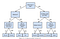Source: Data Science from Scratch: First Principles with Python

This is exactly how we would create a Decision Tree for any Data Science Problem also. Now let us study in detail the math behind it.

# What is a Decision Tree?

• Credit scoring models in which the criteria that cause an applicant to be rejected need to be clearly documented and free from bias
• Marketing studies of customer behavior such as satisfaction or churn, which will be shared with management or advertising agencies
• Diagnosis of medical conditions based on laboratory measurements, symptoms, or the rate of disease progression

# Structure of a Decision Tree

• Root Node: The node that performs the first split. In the above “Guess the Animal” example, the root node would be the question `lives in water.`
• Terminal Nodes/Leaves :Nodes that predict the outcome. Likewise, fort he example above, terminal nodes would be `bull ,cow, Lion, Tiger etc`
• Branches: arrows connecting nodes, showing the flow from question to answer.

The root node is the starting point of the tree, and both root and terminal nodes contain questions or criteria to be answered. Each node typically has two or more nodes extending from it. For example, if the question in the first node requires a “yes” or “no” answer, there will be one leaf node for a “yes” response, and another node for “no.”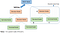PC: Analytics VIdhya

# The Algorithm behind Decision Trees.

• a continuous variable, for regression trees
• a categorical variable, for classification trees

The decision rules generated by the CART (Classification & Regression Trees) predictive model are generally visualized as a binary tree.

Let’s look at an example to understand it better. The plot below shows a sample data for two independent variables, x, and y, and each data point is colored by the outcome variable, red or grey.

CART tries to split this data into subsets so that each subset is as pure or homogeneous as possible. The first three splits that CART would create are shown here.

If a new observation fell into any of the subsets, it would now be decided by the majority of the observations in that particular subset.

Let us now see how a Decision Tree algorithm generates a TREE. The tree for the splits we just generated is shown below.

• The first split tests whether the variable x is less than 60. If yes, the model says to predict red, and if no, the model moves on to the next split.
• Then, the second split checks whether or not the variable y is less than 20.
If no, the model says to predict gray, but if yes, the model moves on to the next split.

The third split checks whether or not the variable x is less than 85. If yes, then the model says to predict red, and if no, the model says to predict grey.

# Advantages of Decision Trees

• It can also be used to identify the most significant variables in your data-set

# Predictions from Decision Trees

## Classification Trees:

Let us understand it through the same example that we used above.

The above dataset has been split into four subsets.

Predictions for Subset 1:

• Red data = 7, Grey data = 2
• % of Red data = 7/(7+2) ~ 78% and % of Grey data ~22%. This means 78% of the data is Red.
• Now just like in Logistic Regression, we can use a threshold value to obtain our prediction.
• A Threshold of 0.5/50%, corresponds to picking most frequent outcome which would be Red.
• But if we increase that threshold to 0.9/90%, we would predict Grey

## Regression Trees:

Let us see it graphically.

In the above graph:

y = Outcome/target variable i.e variable we are trying to predict

x = Independent variable

Firstly, Let’s fit a linear regression to this data set . By doing so , we obtain a line .

As is quite evident, linear regression does not do very well on this data set.

However, we can notice a very interesting feature. The data lies in three different groups. If we draw lines here, we see x is either less than 10, between 10 and 20, or greater then 20.

We recall that Decision Trees can fit in this this kind of of problem easily. So if splits are at:

• x ≤10 |output would be the average of those values.
• 10 < x ≤ 20 |output would be the average of those values.
• 20< x≤ 30 |output would be the average of those values.

# Measures Used for Split

1. Gini Index: It is the measure of inequality of distribution. It says if we select two items from a population at random then they must be of same class and probability for this is 1 if population is pure.
• It works with categorical target variable “Success” or “Failure”.
• It performs only Binary splits
• Lower the value of Gini, higher the homogeneity.
• CART uses Gini method to create binary splits.

Process to calculate Gini Measure:

where P(j) is the Probability of Class j

2. Entropy : Entropy is a way to measure impurity.

Less impure node require less information to describe them and more impure node require more information. If the sample is completely homogeneous, then the entropy is zero and if the sample is an equally divided one, it has entropy of one.

3. Information Gain : Information Gain is simply a mathematical way to capture the amount of information one gains(or reduction in randomness) by picking a particular attribute.

In a decision algorithm, we start at the tree root and split the data on the feature that results in the largest information gain (IG). In other words, IG tells us how important a given attribute is.

The Information Gain (IG) can be defined as follows:

Where I could be entropy or Gini index. D(p), D(Left) and D(Right) are the dataset of the parent, left and right child node.

In R, a parameter that controls this is minbucket. The smaller it is, the more splits will be generated However, If it is too small, overfitting will occur. And, if it is too large, model will be too simple and accuracy will be poor

# Decision Trees in R

## Dataset

`LON and LAT are the longitude and latitude of the center of the census tract.MEDV is the median value of owner-occupied homes, measuredin thousands of dollars.CRIM is the per capita crime rate.ZN is related to how much of the land is zoned for large residential properties.INDUS is the proportion of the area used for industry.CHAS is 1 if a census tract is next to the Charles River else 0 NOX is the concentration of nitrous oxides in the air, a measure of air pollution.RM is the average number of rooms per dwelling.AGE is the proportion of owner-occupied units built before 1940.DIS is a measure of how far the tract is from centres of employment in Boston.RAD is a measure of closeness to important highways.TAX is the property tax per \$10,000 of value.PTRATIO is the pupil to teacher ratio by town.`

here MEDV is the output /target variable i.e price of the house to be predicted. Since the output variable is continuous it is an example of a regression tree.

# Working

## 1. Analyzing the Data

`> boston = read.csv('boston.csv')> str(boston)'data.frame': 506 obs. of  16 variables: \$ TOWN   : Factor w/ 92 levels "Arlington","Ashland",..: 54 77 77 46 46 46 69 69 69 69 ... \$ TRACT  : int  2011 2021 2022 2031 2032 2033 2041 2042 2043 2044 ... \$ LON    : num  -71 -71 -70.9 -70.9 -70.9 ... \$ LAT    : num  42.3 42.3 42.3 42.3 42.3 ... \$ MEDV   : num  24 21.6 34.7 33.4 36.2 28.7 22.9 22.1 16.5 18.9 ... \$ CRIM   : num  0.00632 0.02731 0.02729 0.03237 0.06905 ... \$ ZN     : num  18 0 0 0 0 0 12.5 12.5 12.5 12.5 ... \$ INDUS  : num  2.31 7.07 7.07 2.18 2.18 2.18 7.87 7.87 7.87 7.87 ... \$ CHAS   : int  0 0 0 0 0 0 0 0 0 0 ... \$ NOX    : num  0.538 0.469 0.469 0.458 0.458 0.458 0.524 0.524 0.524 0.524 ... \$ RM     : num  6.58 6.42 7.18 7 7.15 ... \$ AGE    : num  65.2 78.9 61.1 45.8 54.2 58.7 66.6 96.1 100 85.9 ... \$ DIS    : num  4.09 4.97 4.97 6.06 6.06 ... \$ RAD    : int  1 2 2 3 3 3 5 5 5 5 ... \$ TAX    : int  296 242 242 222 222 222 311 311 311 311 ... \$ PTRATIO: num  15.3 17.8 17.8 18.7 18.7 18.7 15.2 15.2 15.2 15.2 ...`

There are 506 observations corresponding to 506 census tracts in the Greater Boston area. We are interested in building a model of how prices vary by location across a region. So, let’s first see how the points are laid out.
Using the plot commands, we can plot the latitude and longitude
of each of our census tracts.

Let’s first see how the points are laid out using the plot commands.

`# Plot observations> plot(boston\$LON, boston\$LAT)`

The dense central core of points
corresponds to Boston city, and other urban cities.
Since, we also have the Charles river attribute(CHAS), we want to also show all the points that lie along the Charles River in a blue colour.

We can do this by the points command.

`# Tracts alongside the Charles River> points(boston\$LON[boston\$CHAS==1], boston\$LAT[boston\$CHAS==1], col="blue", pch=19)`

Now we have plotted the tracks in Boston along Charles river.

What other things can we do?

Well, this data set was originally constructed to investigate questions about how air pollution affects prices.
The air pollution variable in the data is NOX. Let’s have a look at a distribution of NOX.

`# Plot pollution/NOX > summary(boston\$NOX) Min. 1st Qu.  Median    Mean 3rd Qu.    Max.  0.3850  0.4490  0.5380  0.5547  0.6240  0.8710`

The minimum value is 0.385 and the maximum value is 0.87 .The median
and the mean are about 0.53, 0.55. So, let’s just use the value of 0.55,
as it is the centre most value.

Let’s look at the tracts that have above-average pollution.

`> points(boston\$LON[boston\$NOX>=0.55], boston\$LAT[boston\$NOX>=0.55], col="green", pch=20)`

All the points that have got above-average pollution are coloured green.
Now it kind of makes sense, since, the area most densely polluted is the one which is also most densely populated.

Now let us look at how the prices vary over the area as well. We can do this with the help of MEDV variable using the same methodology as done when plotting the pollution.

`# Plot prices> plot(boston\$LON, boston\$LAT)> summary(boston\$MEDV)   Min. 1st Qu.  Median    Mean 3rd Qu.    Max.    5.00   17.02   21.20   22.53   25.00   50.00> points(boston\$LON[boston\$MEDV>=21.2], boston\$LAT[boston\$MEDV>=21.2], col="red", pch=20)`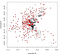above median values graph

So what we see now are all the census tracts with above-average housing prices in red.
However, the census tracts of above-average and below-average are mixed in between each other.
But there are some patterns.
For example, look at that dense black bit in the middle. That corresponds to most of the city of Boston, especially the southern parts of the city.
So there’s definitely some structure to it, but it’s certainly not simple in relation to latitude and longitude at least.

## 2. Applying Linear Regression to the problem

Here we are plotting the relationship between latitude and house
prices
and the longitude and the house prices, which looks pretty nonlinear.

`# Linear Regression using LAT and LON> plot(boston\$LAT, boston\$MEDV)> plot(boston\$LON, boston\$MEDV)`

## Linear Regression Model

`> latlonlm = lm(MEDV ~ LAT + LON, data=boston)> summary(latlonlm)Call:lm(formula = MEDV ~ LAT + LON, data = boston)Residuals:    Min      1Q  Median      3Q     Max -16.460  -5.590  -1.299   3.695  28.129Coefficients:             Estimate Std. Error t value Pr(>|t|)    (Intercept) -3178.472    484.937  -6.554 1.39e-10 ***LAT             8.046      6.327   1.272    0.204    LON           -40.268      5.184  -7.768 4.50e-14 ***---Signif. codes:  0 '***' 0.001 '**' 0.01 '*' 0.05 '.' 0.1 ' ' 1Residual standard error: 8.693 on 503 degrees of freedomMultiple R-squared:  0.1072, Adjusted R-squared:  0.1036 F-statistic: 30.19 on 2 and 503 DF,  p-value: 4.159e-13`
• R-squared is around 0.1, which is not great.
• The latitude is not significant, which means the north-south location differences aren’t going to be really used at all. This also seems unlikely.
• Longitude is significant, but negative which means that as we go towards the east house prices decrease linearly, which is also unlikely.

Let’s see how this linear regression model looks on a plot.So we shall plot the census tracts again and then plot the above-median house prices with bright red dots. The red dots will tell us the actual positions in Boston where houses are costly. We shall then test the same fact with Linear Regression predictions using blue \$ sign,

`# Visualize regression output> plot(boston\$LON, boston\$LAT)> points(boston\$LON[boston\$MEDV>=21.2], boston\$LAT[boston\$MEDV>=21.2], col="red", pch=20)> latlonlm\$fitted.values> points(boston\$LON[latlonlm\$fitted.values >= 21.2], boston\$LAT[latlonlm\$fitted.values >= 21.2], col="blue", pch="\$")`

The linear regression model has plotted a dollar sign for every time it thinks the census tract is above median value. It’s almost a sharp line that the linear regression defines. Also notice the shape is almost vertical since the latitude variable was not very significant in the regression. The blue \$ and the red dots do not overlap especially in the east.

It turns out, the linear regression model isn’t really doing a good job. And it has completely ignored everything to the right side of the picture. So that’s interesting and pretty wrong.

## 3. Applying Regression Trees to the problem

`# Load CART packages> library(rpart)# install rpart package> install.packages("rpart.plot")> library(rpart.plot)`

We will build a regression tree in the same way we would build a classification tree, using the rpart command.We would be predicting `MEDV` as a function of latitude and longitude, using the `boston `dataset.

`# CART model> latlontree = rpart(MEDV ~ LAT + LON, data=boston)# Plot the tree using prp command defined in rpart.plot package> prp(latlontree)`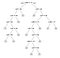Regression Tree

The leaves of the tree are important.

• In a classification tree, the leaves would be the classification that we assign .
• In regression trees, we instead predict the number.That number here is the average of the median house prices in that bucket.

Now, Let us visualise the output.We’ll again plot the points with above median prices just like in Linear Regression

`# Visualize output> plot(boston\$LON, boston\$LAT)>points(boston\$LON[boston\$MEDV>=21.2],boston\$LAT[boston\$MEDV>=21.2], col="red", pch=20)`

The above plot is of actual known prices.It is the same plot that we observed with red dots. We want to predict what the tree thinks is above median house price. So we’ll name those values as fitted values which can be obtained from using the predict command on the tree we just built.

`> fittedvalues = predict(latlontree)>points(boston\$LON[fittedvalues>21.2],boston\$LAT[fittedvalues>=21.2], col="blue", pch="\$")`

The fitted values are greater than 21.2, the colour is blue, and the character is a \$ to signify Price

The Regression tree has done a much better job and has kind of overlapped the red dots. It has left the low value area in Boston
out, and has correctly managed to classify some of those points
in the bottom right and top right.
`

But the tree obtained was very complicated and was overfitted.
How to avoid overfitting? By changing the `minbucket` size.
So let’s build a new tree using the `rpart` command again.

## Simplifying Tree by increasing minbucket

`> latlontree = rpart(MEDV ~ LAT + LON, data=boston, minbucket=50)> plot(latlontree)> text(latlontree)`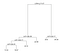tree with minbucket = 50

Here we have far fewer splits, and it’s far more interpretable.

We have seen that regression trees can do that we would never expect linear regression to do. Now let’s see how the regression trees will help us in building a predictive model and predicting house prices?

## 4. Prediction with Regression Trees

Steps:

• Split the data into Training and Testing set using `caTools` library. We shall then set the seed so our results are reproducible.
`# Split the data> library(caTools)> set.seed(123)> split = sample.split(boston\$MEDV, SplitRatio = 0.7)> train = subset(boston, split==TRUE)> test = subset(boston, split==FALSE)`

Our training data is a subset of the boston data where the split is TRUE.
And the testing data is the subset of the boston data where the split is FALSE

• Making a Regression Tree Model
`# Create a CART model> tree = rpart(MEDV ~ LAT + LON + CRIM + ZN + INDUS + CHAS + NOX + RM + AGE + DIS + RAD + TAX + PTRATIO, data=train)> prp(tree)`

## Results:

• The rooms are the most important split.
• Pollution appears in there twice, so it’s, in some sense, nonlinear on the amount of pollution i.e if it’s greater than a certain amount or less than a certain amount, it does different things.
• Very nonlinear on the number of rooms.

## Regression Tree Predictions

`> tree.pred = predict(tree, newdata=test)> tree.sse = sum((tree.pred - test\$MEDV)^2)> tree.sse4328.988`

# Conclusion

There are techniques which help in improving the performance of Decision Trees but we will learn about them but in the next article. We will learn to improve the results of our Decision Trees using the “cp” parameter. Also, we will try and implement Cross Validation, a technique to avoid overfitting in predictive models. Finally we will dive into Ensembling i.e combining the results of multiple models to solve a given prediction or classification problem.

So stay tuned for the next part.

## Analytics Vidhya

Analytics Vidhya is a community of Analytics and Data…

Thanks to Prateek Joshi

## Sign up for Analytics Vidhya News Bytes

### By Analytics Vidhya

Latest news from Analytics Vidhya on our Hackathons and some of our best articles! Take a look.

By signing up, you will create a Medium account if you don’t already have one. Review our Privacy Policy for more information about our privacy practices.

Check your inbox
Medium sent you an email at to complete your subscription.

Written by

## Parul Pandey

Data Science @H2O.ai | Editor @wicds

## Analytics Vidhya

Analytics Vidhya is a community of Analytics and Data Science professionals. We are building the next-gen data science ecosystem https://www.analyticsvidhya.com

Written by

## Parul Pandey

Data Science @H2O.ai | Editor @wicds## Analytics Vidhya

Analytics Vidhya is a community of Analytics and Data Science professionals. We are building the next-gen data science ecosystem https://www.analyticsvidhya.com

## More From Medium

Medium is an open platform where 170 million readers come to find insightful and dynamic thinking. Here, expert and undiscovered voices alike dive into the heart of any topic and bring new ideas to the surface. Learn more

Follow the writers, publications, and topics that matter to you, and you’ll see them on your homepage and in your inbox. Explore

If you have a story to tell, knowledge to share, or a perspective to offer — welcome home. It’s easy and free to post your thinking on any topic. Write on Medium